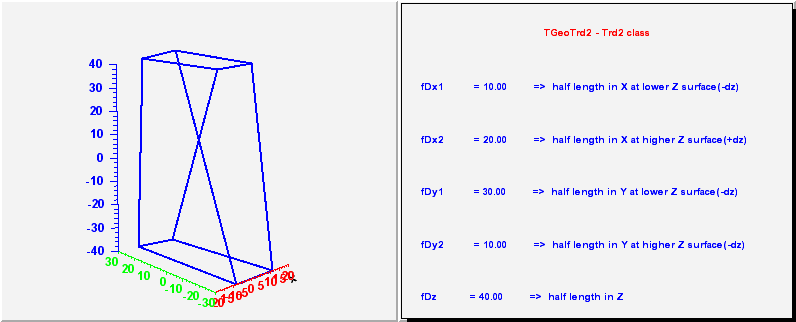library: libGeom#include "TGeoTrd2.h"

# TGeoTrd2

class description - source file - inheritance tree (.pdf)

## class TGeoTrd2 : public TGeoBBox

Inheritance Chart:
 TObject
<-
TNamed
<-
TGeoShape
<-
TGeoBBox
<-
TGeoTrd2
```
public:
TGeoTrd2()
TGeoTrd2(Double_t dx1, Double_t dx2, Double_t dy1, Double_t dy2, Double_t dz)
TGeoTrd2(const char* name, Double_t dx1, Double_t dx2, Double_t dy1, Double_t dy2, Double_t dz)
TGeoTrd2(Double_t* params)
TGeoTrd2(const TGeoTrd2&)
virtual ~TGeoTrd2()
static TClass* Class()
virtual void ComputeBBox()
virtual void ComputeNormal(Double_t* point, Double_t* dir, Double_t* norm)
virtual Bool_t Contains(Double_t* point) const
virtual Double_t DistFromInside(Double_t* point, Double_t* dir, Int_t iact = 1, Double_t step = TGeoShape::Big(), Double_t* safe = 0) const
virtual Double_t DistFromOutside(Double_t* point, Double_t* dir, Int_t iact = 1, Double_t step = TGeoShape::Big(), Double_t* safe = 0) const
virtual TGeoVolume* Divide(TGeoVolume* voldiv, const char* divname, Int_t iaxis, Int_t ndiv, Double_t start, Double_t step)
virtual Double_t GetAxisRange(Int_t iaxis, Double_t& xlo, Double_t& xhi) const
virtual void GetBoundingCylinder(Double_t* param) const
virtual Int_t GetByteCount() const
Double_t GetDx1() const
Double_t GetDx2() const
Double_t GetDy1() const
Double_t GetDy2() const
Double_t GetDz() const
virtual Int_t GetFittingBox(const TGeoBBox* parambox, TGeoMatrix* mat, Double_t& dx, Double_t& dy, Double_t& dz) const
virtual TGeoShape* GetMakeRuntimeShape(TGeoShape* mother, TGeoMatrix* mat) const
virtual Int_t GetNmeshVertices() const
void GetOppositeCorner(Double_t* point, Int_t inorm, Double_t* vertex, Double_t* normals) const
void GetVisibleCorner(Double_t* point, Double_t* vertex, Double_t* normals) const
virtual void InspectShape() const
virtual TClass* IsA() const
virtual Bool_t IsCylType() const
TGeoTrd2& operator=(const TGeoTrd2&)
virtual Double_t Safety(Double_t* point, Bool_t in = kTRUE) const
virtual void SetDimensions(Double_t* param)
virtual void SetPoints(Double_t* buff) const
virtual void SetPoints(Float_t* buff) const
void SetVertex(Double_t* vertex) const
virtual void ShowMembers(TMemberInspector& insp, char* parent)
virtual void Sizeof3D() const
virtual void Streamer(TBuffer& b)
void StreamerNVirtual(TBuffer& b)
```

### Data Members

```
protected:
Double_t fDx1  half length in X at lower Z surface (-dz)
Double_t fDx2  half length in X at higher Z surface (+dz)
Double_t fDy1  half length in Y at lower Z surface (-dz)
Double_t fDy2  half length in Y at higher Z surface (+dz)
Double_t fDz   half length in Z
```

## Class Description

``` TGeoTrd2 - a trapezoid with both x and y lengths varying with z. It
has 5 parameters, the half lengths in x at -dz and +dz, the half
lengths in y at -dz and +dz, and the half length in z (dz).

_____________________________________________________________________________
```
/**/

TGeoTrd2()
``` dummy ctor
```

TGeoTrd2(Double_t dx1, Double_t dx2, Double_t dy1, Double_t dy2, Double_t dz) :TGeoBBox(0,0,0)
``` constructor.
```

TGeoTrd2(const char * name, Double_t dx1, Double_t dx2, Double_t dy1, Double_t dy2, Double_t dz) :TGeoBBox(name, 0,0,0)
``` constructor.
```

TGeoTrd2(Double_t *param) :TGeoBBox(0,0,0)
``` ctor with an array of parameters
param = dx1
param = dx2
param = dy1
param = dy2
param = dz
```

~TGeoTrd2()
``` destructor
```

void ComputeBBox()
``` compute bounding box for a trd2
```

void ComputeNormal(Double_t *point, Double_t *dir, Double_t *norm)
``` Compute normal to closest surface from POINT.
```

Bool_t Contains(Double_t *point) const
``` test if point is inside this shape
check Z range
```

Double_t DistFromInside(Double_t *point, Double_t *dir, Int_t iact, Double_t step, Double_t *safe) const
``` compute distance from inside point to surface of the trd2
```

Double_t DistFromOutside(Double_t *point, Double_t *dir, Int_t iact, Double_t step, Double_t *safe) const
``` compute distance from outside point to surface of the trd2
```

Double_t GetAxisRange(Int_t iaxis, Double_t &xlo, Double_t &xhi) const
``` Get range of shape for a given axis.
```

void GetVisibleCorner(Double_t *point, Double_t *vertex, Double_t *normals) const
``` get the most visible corner from outside point and the normals
```

void GetOppositeCorner(Double_t * /*point*/, Int_t inorm, Double_t *vertex, Double_t *normals) const
``` get the opposite corner of the intersected face
```

TGeoVolume* Divide(TGeoVolume *voldiv, const char *divname, Int_t iaxis, Int_t ndiv, Double_t start, Double_t step)
```--- Divide this trd2 shape belonging to volume "voldiv" into ndiv volumes
called divname, from start position with the given step. Only Z divisions
are supported. For Z divisions just return the pointer to the volume to be
divided. In case a wrong division axis is supplied, returns pointer to
volume that was divided.
```

void GetBoundingCylinder(Double_t *param) const
```--- Fill vector param with the bounding cylinder parameters. The order
is the following : Rmin, Rmax, Phi1, Phi2
```

Int_t GetFittingBox(const TGeoBBox *parambox, TGeoMatrix *mat, Double_t &dx, Double_t &dy, Double_t &dz) const
``` Fills real parameters of a positioned box inside this. Returns 0 if successfull.
```

TGeoShape* GetMakeRuntimeShape(TGeoShape *mother, TGeoMatrix * /*mat*/) const
``` in case shape has some negative parameters, these has to be computed
in order to fit the mother
```

void InspectShape() const
``` print shape parameters
```

Double_t Safety(Double_t *point, Bool_t in) const
``` computes the closest distance from given point to this shape, according
to option. The matching point on the shape is stored in spoint.
```

void SetDimensions(Double_t *param)
``` set arb8 params in one step :
```

void SetPoints(Double_t *buff) const
``` create trd2 mesh points
```

void SetPoints(Float_t *buff) const
``` create trd2 mesh points
```

void SetVertex(Double_t *vertex) const
``` set vertex of a corner according to visibility flags
```

void Sizeof3D() const
``` fill size of this 3-D object
```

### Inline Functions

```              Int_t GetByteCount() const
Double_t GetDx1() const
Double_t GetDx2() const
Double_t GetDy1() const
Double_t GetDy2() const
Double_t GetDz() const
Int_t GetNmeshVertices() const
Bool_t IsCylType() const
TClass* Class()
TClass* IsA() const
void ShowMembers(TMemberInspector& insp, char* parent)
void Streamer(TBuffer& b)
void StreamerNVirtual(TBuffer& b)
TGeoTrd2 TGeoTrd2(const TGeoTrd2&)
TGeoTrd2& operator=(const TGeoTrd2&)
```

Author: Andrei Gheata 31/01/02
Last update: root/geom:\$Name: \$:\$Id: TGeoTrd2.cxx,v 1.25 2004/11/08 09:56:24 brun Exp \$